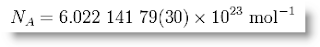## Friday, June 26, 2009

Wikipedia's entry on the Mole, a measure of substance, complains that "the [misconception that the] mole is simply a counting aid, has even found its way into elementary chemistry textbooks". That's good cause for worry, as what gets into elementary works tends to have a long half life sometimes.

In any case, we do have Avogadro's Number as the number of carbon atoms in 12 grams of carbon-12, and yes that sounds stupid and arbitrary but at least 12 is easily divisible by a lot of factors, unlike 10 with just two factors.Suppose you have a cuboctahedron of cee cells, those mythical rhombic dodecahedral casements built by "cees" instead of "bees". It's a "ghost ship" in that, like a grid of XYZ cubes, it has only ethereal existence as a "grid" i.e. doesn't disturb the waters with any eddies or ionization.

Say we're floating our inert assembly in an ideal gas of uniform density and want to fine tune our shape to contain one Mole of said substance i.e. an average of one molecule at the center of each cell. What would be the frequency of said cuboctahedron?

A brute force approach would be to simply accumulate a running total on our old friend, the number sequence 1, 12, 42, 92... The running total there is 1, 13, 55, 147... where frequency is 0, 1, 2, 3... etc. Let's "do the math":

Usefully, we might now actually attach a real scale to the frequency 56,531,530, to compute any one of the edges of our 14 windowed, 12 cornered reference shape. That number times the interval between any two ball centers (gas molecules) in the CCP will give you an empirical volume.

It's not like the gas molecules are really "standing still" nor does our reference shape exert pressure. Think of the gas containment as relatively huge, walls unseen, and taking this "core sample" (like a biopsy) somewhere towards the middle, where conditions are settled and uniform (i.e. "rank and file" from a statistical standpoint).

The cuboctahedron has some advantages over the cube, e.g. equal circumferential and radial intervals, but if you prefer to work in cubes, just remember the 20:3 ratio.